×#### Thank you for registering.

One of our academic counsellors will contact you within 1 working day.

Click to Chat

1800-1023-196

+91-120-4616500

CART 0

• 0

MY CART (5)

Use Coupon: CART20 and get 20% off on all online Study Material

ITEM
DETAILS
MRP
DISCOUNT
FINAL PRICE
Total Price: Rs.

There are no items in this cart.
Continue Shopping```Operations on Determinants

Multiplication of two DeterminantsTwo determinants can be multiplied together only if they are of same order. The rule of multiplication is as under:

Take the first row of determinant and multiply it successively with 1st, 2nd & 3rd rows of other determinant. The three expressions thus obtained will be elements of 1st row of resultant determinant. In a similar manner the element of 2nd & 3rd rows of determinant are obtained.where, R1 → first row of first determinant

Ri  → first row of second determinant

Illustration:

Find the product of the determinants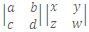Solution:

=Differentiation of a Determinant

Yes, let f(x) =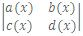be a given function.

=> f(x) = a(x) d(x) - c(x) b(x)

=> f'(x) = a'(x) d(x) + a(x) d'(x) - c'(x) b(x) - c(x) b'(x)

=> a'(x) d(x) - c'(x) b(x) + a(x) d'(x) - c(x) b'(x)

=Thus, the differential coefficient of a determinant is obtained by differentiating a single row (or column) at a time and finally adding the determinants so obtained.

Thus, for a determinant of order 'n'.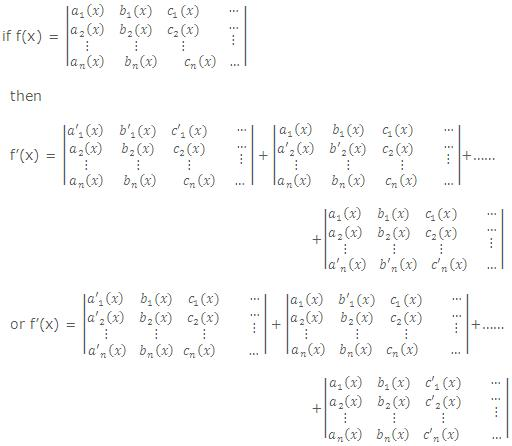Summation of Determinants

Let Δr=, where a,b,c,l,m and n are constants independents of r,

then  =∑nr=1  ?r =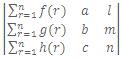Here functions of r can be the elements of only one row or column. None of the elements other of than that row or column should be dependent on r.

Illustration:

Let Δa=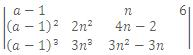. Showthat ∑na=1 ?a = 0

Solution:

By adding all the corresponding elements of C1 of all determinants Δa we have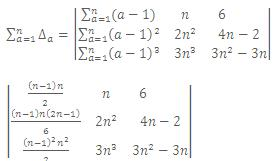By taking (n-1)n/2 as common factor from C1 and 6 as common factor from C3, we get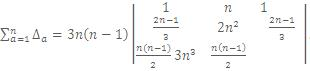Since C1 and C3 are identical ∑na=1  ?a =0.

Special Determinants

1. Symmetric determinant

The elements situated at equal distance from the diagonal are equal both in magnitude and sign.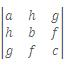= abc + 2fgh - af2 - bg2 - ch2.

2. Skew symmetric determinant

All the diagonal elements are zero and the elements situated at equal distance form the diagonal are equal in magnitude but opposite in sign. The value of a skew symmetric determinant of odd order is zero.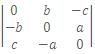= 0.

3. Circulant determinant:

The elements of the rows (or columns) are in cyclic arrangement.= -(a3 + b3 + c3 - 3abc).

4.= (a-b)(b-c)(c-a).

5.= (a-b)(b-c)(c-a)(a+b+c).

6.= (a-b)(b-c)(c-a)(ab+bc+ca).

Be a participant in the online tests conducted by askIITians and also give answers in AQAD ( A Question  A Day) get a number of benefits in the online live classroom courses and also read online Study material for IIT JEE and JEE Main /Advanced (known as AIEEE till 2012) preparation absolutely free.

To read more, Buy study materials of Matrices and Determinants comprising study notes, revision notes, video lectures, previous year solved questions etc. Also browse for more study materials on Mathematics here.
```### Course Features

• 731 Video Lectures
• Revision Notes
• Previous Year Papers
• Mind Map
• Study Planner
• NCERT Solutions
• Discussion Forum
• Test paper with Video Solution